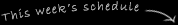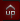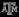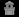#Math Tutoring in Bryan College Station Texas

A+ Tutoring currently offers math tutoring in classroom for the classes listed below. Course numbers follow Texas A&M University's course numbering system; where available, the corresponding math course number for Blinn College is indicated below the class description. (Please see our courses page for current availability of private tutoring for math classes.)##MATH 131 Tutoring (“Mathematical Concepts - Calculus”)

Official Texas A&M University course description for MATH 131: Limits and continuity; rates of change, slope; differentiation: the derivative, maxima and minima; integration: the definite and indefinite integral techniques; curve fitting.

##MATH 141 Tutoring (“Business Mathematics I”)

Official Texas A&M University course description for MATH 141: Linear equations and applications, systems of linear equations, matrix algebra and applications, linear programming (graphical and simplex methods), probability and applications, statistics.

TAMU class MATH 141 corresponds to Blinn College math course MATH 1324 (“MATH, ANALYSIS I”)

##MATH 142 Tutoring (“Business Mathematics II”)

Official Texas A&M University course description for MATH 142: Derivatives, curve sketching and optimization, techniques of derivatives, logarithms and exponential functions with applications, integrals, techniques and applications of integrals, multivariate calculus.

TAMU class MATH 142 corresponds to Blinn College math course MATH 1325 (“MATH, ANALYSIS II”)

##MATH 151 Tutoring (“Engineering Mathematics I”)

Official Texas A&M University course description for MATH 151: Rectangular coordinates, vectors, analytic geometry, functions, limits, derivatives of functions, applications, integration, computer algebra (Maple).

TAMU class MATH 151 corresponds to Blinn College math course MATH 2413 (“CALCULUS I (DIFFERENTIAL)”)

##MATH 152 Tutoring (“Engineering Mathematics II”)

Official Texas A&M University course description for MATH 152: Differentiation and integration techniques and their applications (area, volumes, work), improper integrals, approximate integration, analytic geometry, vectors, infinite series, power series, Taylor series, computer algebra (Maple).

TAMU class MATH 152 corresponds to Blinn College math course MATH 2414 (“CALCULUS II (INTEGRAL)”)

##MATH 166 Tutoring (“Topics in Contemporary Mathematics II”)

Official Texas A&M University course description for MATH 166: Finite mathematics, matrices, probability and applications.

##MATH 171 Tutoring (“Analytic Geometry and Calculus”)

Official Texas A&M University course description for MATH 171: Vectors, functions, limits, derivatives, Mean Value Theorem, applications of derivatives, integrals, Fundamental Theorem of Calculus.

##MATH 172 Tutoring (“Calculus”)

Official Texas A&M University course description for MATH 172: Techniques of integration, applications of integrals, improper integrals, sequences, infinite series, vector algebra and solid analytic geometry.

##MATH 251 Tutoring (“Engineering Mathematics III”)

Official Texas A&M University course description for MATH 251: Vector algebra, calculus of functions of several variables, partial derivatives, directional derivatives, gradient, multiple integration, line and surface integrals, Stokes' theorems.

##MATH 253 Tutoring (“Engineering Mathematics III”)

Official Texas A&M University course description for MATH 253: Vector algebra; calculus of functions of several variables, partial derivatives, directional derivatives, gradient, multiple integration, line and surface integrals, Green’s and Stokes’ theorems, computer algebra.

TAMU class MATH 253 corresponds to Blinn College math course MATH 2415 (“CALCULUS III”)

##MATH 308 Tutoring (“Differential Equations”)

Official Texas A&M University course description for MATH 308: Ordinary differential equations, solutions in series, solutions using Laplace transforms, systems of differential equations.College Station Web DesignCopyright © 2020 A+ Tutoring in Bryan College Station (Aggieland) — class tutoring and some private tutoring for Texas A&M Aggies and Blinn College students